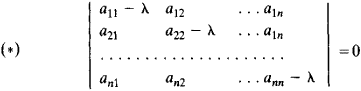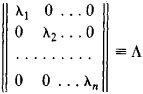# eigenvalue

Also found in: Dictionary, Thesaurus, Medical, Wikipedia.
Related to eigenvalue: eigenvalue equation, Eigenvalue Problem

## Eigenvalue (quantum mechanics)

If an equation containing a variable parameter possesses nontrivial solutions only for certain special values of the parameter, these solutions are called eigenfunctions and the special values are called eigenvalues.

The eigenfunction-eigenvalue relation is of particular importance in quantum mechanics because of its prominence in the equations which relate the mathematical formalism of the theory with physical results. See Quantum mechanics

McGraw-Hill Concise Encyclopedia of Physics. © 2002 by The McGraw-Hill Companies, Inc.
The following article is from The Great Soviet Encyclopedia (1979). It might be outdated or ideologically biased.

## Eigenvalue

(or characteristic value). An eigenvalue of a linear transformation or operator A is a number λ for which there exists a nonzero vector x such that Ax = λx; the vector x is called an eigenvector, or characteristic vector. Thus, the eigenvalues of a differential operator L(y) with given boundary conditions are numbers X for which the equation L(y) = λy has a nonzero solution that satisfies the boundary conditions. For example, if the operator L(y) has the form -y”, then numbers of the form λn = n2, where n is a natural number, are eigenvalues of the operator under the boundary conditions y (0) = y (π) = 0, since the functions yn = sin nx satisfy the equation - yn = n2y with the indicated boundary conditions. If, however, λ + n2 for any natural n, then only the function y(x) = 0 satisfies the equation –y” = λy under the same boundary conditions. Eigenvalues of linear operators are of importance in many problems in mathematics, mechanics, and physics—in problems, for example, in analytic geometry, algebra, the theory of vibrations, and quantum mechanics.

The eigenvalues of the matrix A = ║aik║, where i, k = 1, 2, . . . , n, are the eigenvalues of the linear transformation on an n-dimensional complex space that corresponds to A. The eigenvalues can also be defined as the roots of the equation det(A - λE) = 0, where E is the unit matrix—that is, the roots of the equationwhich is called the characteristic equation of the matrix. Since these numbers are the same for the similar matrices A and B’XAB where B is a nonsingular matrix, they characterize properties of the linear transformation that are independent of the choice of coordinate system. To each root λ, of equation (*) there corresponds a vector xi ≠ 0 (an eigenvector) such that Axi = λixi. If all the eigenvalues are distinct, then the set of eigenvectors may be chosen as the basis of the vector space. With respect to this basis, the linear transformation is described by the diagonal matrixEvery matrix A with distinct eigenvalues can be represented in the form C–1ʌC. If A is a Hermitian matrix, then its eigenvalues are real, the eigenvectors are orthogonal, and there exists a unitary matrix that can be chosen as C. The absolute value of every eigenvalue of a unitary matrix is equal to 1. The sum of the eigenvalues of a matrix is equal to the sum of its diagonal elements—that is, to the trace of the matrix. Knowledge of the eigenvalues of a matrix plays an important role in the investigation of the convergence of certain approximate methods of solving systems of linear equations.

## eigenvalue

[′ī·gən‚val·yü]
(mathematics)
The one of the scalars λ such that T (v) = λ v, where T is a linear operator on a vector space, and v is an eigenvector. Also known as characteristic number; characteristic root; characteristic value; latent root; proper value.
McGraw-Hill Dictionary of Scientific & Technical Terms, 6E, Copyright © 2003 by The McGraw-Hill Companies, Inc.

## eigenvalue

(mathematics)
The factor by which a linear transformation multiplies one of its eigenvectors.
References in periodicals archive ?
Then the fact that all eigenvalues of A are inside the set restricted by the figure [mathematical expression not reproducible] guarantees system (5) (or equivalently, (6) or (10)) to be asymptotically stable.
In Section 4, generalized eigenvalue problems are formed on faces and edges to select the effective adaptive primal constraints which make the resulting algorithms robust to the heterogeneous coefficients.
Let V be a matrix of [L.sup.-1]'s sequence of eigenvectors ([V.sub.n]) and D a diagonal matrix having [L.sup.-1]'s sequence of eigenvalues ([[lambda].sub.n]), at the diagonal.
In particular, the subset of C of metrics admitting a nonsimple eigenvalue of the Laplacian is a countable union of submanifolds of codimension of at least 1.
Our approach is motivated by Brown and Lin  and Smoller , where the infimum of Rayleigh quotient is used to characterize the principal eigenvalues for diverse linear eigenvalue problems of elliptic equations with infinite weight.
Nevertheless, we can say that (26) can be used as an eigenvalue formula of the Schrodinger equation in case of Cornell potential, for practical purposes.
There exists a [lambda] [member of] R such that [H.sub.0] + [lambda][W.sub.n] has an eigenvalue in ([E.sub.1], [E.sub.2]).
If matrix B is not singular they would have to determine eigenspace of the matrix A, to the eigenvalue zero.
Then there exists the number [[alpha].sub.k] [member of] R such that for all [alpha] > [[alpha].sub.k] the eigenvalue [[lambda].sub.k]([alpha]) is a differentiable function and
we know [P.sup.T]P is a symmetric matrix; since P is invertible, we deduce that all the eigenvalues of the matrix [P.sup.T]P are positive real numbers and [P.sup.T]P is positive definite matrix.
The slow feedback gain [K.sub.sr,2] can be obtained using the eigenvalue assignment; that is,
then the fractional Sturm-Liouville eigenvalue problem (3) can be written as

Site: Follow: Share:
Open / Close